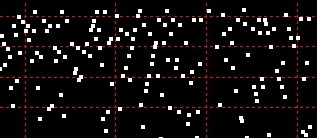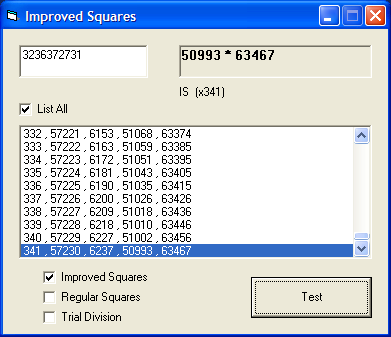exploring the undesigned
intelligence of the numberverse

Lists:Perfect Squares, Composites, Primeshome   Fixing Fermat’s Factorization Method

First published November 8, 2009

The problem with Fermat's factorization method is that it requires, on average, many more iterations than does simple trial division. However, it turns out that there's a simple fix for this.

Fermat's factorization method says that by adding N to perfect squares (PSs) one will find eventually a sum N + PS that is itself a perfect square. When that happens one can know the factors of N by adding and subtracting the square roots of PS and N + PS.

First, one must ask "why use perfect squares at all?" if the factoring is ultimately done with the square roots of the perfect squares.

So let's consider for a moment how we apply this method to a composite with two prime factors (a semiprime):

Say N = 32951

If we begin with 1 + 32951 and continue through all the perfect squares (2*2 , 3*3, 4*4 and so on), we will find that 24649 (157*157) + 32951 finally equals another perfect square: 57600 (240*240).

So 24649 + 32951 = 57600

at which point we know that

240 + 157 = 397
240 - 157 = 83

and that

83 * 397 = 32951

All well and good, but factoring 32951 this way requires evaluating 157 perfect squares.

Can we speed this up? It turns out, Yes! Not just a bit but by a factor of 3 at least....

We know that if we're adding perfect squares to N, then if we are to begin the calculation without using perfect squares we would need to add integer square roots (counting numbers) to N - and, if we do that, we must begin with the square root of N.

The square root of 32951 = 181.52410308275868189567916655919

Needless to say, it's not a perfect square root (and, by the way, if it was it would not be solvable using Fermat's factorization method).

Finding a Fix

Once we make the mental leap from perfect squares to perfect square roots, it's apparent that we're considering a counting problem. We need to approach this problem with two ideas in mind.

Idea #1
It's important we start counting and continue counting in the right place.

If we round up the square root to 182 and start counting up and down, we will get to 157 (-25) before 240 (+58) and never find the square roots we're looking for, since one without the other is useless.

So, as we count we need to have continuous 'course corrections' to be at the point midway between the unknown lower square root and the unknown upper square root.

Idea #2
It's important we only count numbers once.

Consider this sequence:

Sqrt(32951 + 196) = 182.063175848385
Sqrt(32951 + 225) = 182.14280112044
Sqrt(32951 + 256) = 182.227879315982
Sqrt(32951 + 289) = 182.318402801253
Sqrt(32951 + 324) = 182.414363469547
Sqrt(32951 + 361) = 182.515752744797
Sqrt(32951 + 400) = 182.622561585364
Sqrt(32951 + 441) = 182.734780488007
Sqrt(32951 + 484) = 182.852399492049
Sqrt(32951 + 529) = 182.975408183723

Adding N to each of the successive perfect squares from 196 to 529 actually changes the square root of the sum by less than 1! And, of course, the bigger N is the greater the number of PSs needed to shift the square root by 1.

Clearly, we only need to evaluate Sqrt(32951 + 196) to know that 33147 is not a perfect square! The remaining 9 calcs are a completely wasted effort.

The following simple algorithm solves both these problems - actually, Step 3 does.

1. Determine the integer square root of N.

sqrN = Int(Sqr(n))

2. Determine the square of Step 1.

sqrN2 = sqrN * sqrN

(Note that sqrN2 is initially the greatest perfect square less than N.)

3. Determine the integer square root of Step 2 minus N.

PCS = Int(Sqr(Abs(sqrN2 - n)))

(Let's say PCS means 'perfected counting square'.)

4. Determine if the sum of Step 2 and Step 3 is a factor of N.

If mod(n, PCS + sqrN) = 0 then we have a factor, and we're done.

(Similarly, PCS minus sqrN would produce the same result.)

5. If not, add 1 to Step 1 (that is, sqrN+1) and repeat.

So how does this method fare with 32951? It reduces the number of required iterations to 59 from 157, as follows:

 Cnt sqrN PCS sqrN-PCS sqrN+PCS 1 182 13 169 195 2 183 23 160 206 3 184 30 154 214 4 185 35 150 220 5 186 40 146 226 6 187 44 143 231 7 188 48 140 236 8 189 52 137 241 9 190 56 134 246 10 191 59 132 250 11 192 62 130 254 12 193 65 128 258 13 194 68 126 262 14 195 71 124 266 15 196 73 123 269 16 197 76 121 273 17 198 79 119 277 18 199 81 118 280 19 200 83 117 283 20 201 86 115 287 21 202 88 114 290 22 203 90 113 293 23 204 93 111 297 24 205 95 110 300 25 206 97 109 303 26 207 99 108 306 27 208 101 107 309 28 209 103 106 312 29 210 105 105 315 30 211 107 104 318 31 212 109 103 321 32 213 111 102 324 33 214 113 101 327 34 215 115 100 330 35 216 117 99 333 36 217 118 99 335 37 218 120 98 338 38 219 122 97 341 39 220 124 96 344 40 221 126 95 347 41 222 127 95 349 42 223 129 94 352 43 224 131 93 355 44 225 132 93 357 45 226 134 92 360 46 227 136 91 363 47 228 137 91 365 48 229 139 90 368 49 230 141 89 371 50 231 142 89 373 51 232 144 88 376 52 233 146 87 379 53 234 147 87 381 54 235 149 86 384 55 236 150 86 386 56 237 152 85 389 57 238 153 85 391 58 239 155 84 394 59 240 157 83 397

The most important thing to notice is the PCS variable:

• It is by definition always half the difference of the potential factors (sqrN-PCS and sqrN+PCS).
• It is increasing in uneven intervals, skipping a lot of numbers to begin with but just a few near the end.
• The PCS variable doesn't just speed up Fermat's method by a factor of 3 or better, it actually makes the method faster than trial division for 'symmetrical semiprimes' of larger magnitudes.*

*OK, I acknowledge that trial div still has the edge for this baby example. Obviously, try something bigger for a fair test, say 9 or 10 digits. Remember that we're interested in the difficult ones - those with only 2 prime factors that are similar in size to the square root. A systematic survey by magnitude will be coming soon.

Now you're ready to try this out for yourself. I've written some simple illustrative code comparing the "Improved Squares" method with the standard method and with trial division.

Instructions

To try this code, you can use any Microsoft Office VBA macro editor, such as for Excel.

Add 1 text box, 1 list box, 1 command button, 2 labels, and 4 checkboxes to make a form like this:Then copy and paste the following code and try to run it...

The results don't lie...!